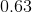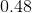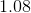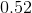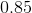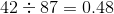## Example Questions

← Previous 1 3

### Example Question #1 : Decimals With Fractions

Simplify: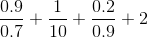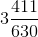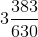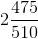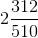Explanation:

1. Convert all of your decimal fractions to normal fractions by multiplying each by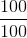: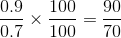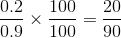2. Find the least common denominator for all of the fractions and use that denominator:

In this case it's 7 x 9 x 10 = 630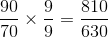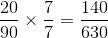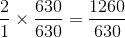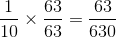3. Add together all of the fractions you now have: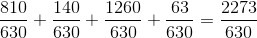4. Simplify this fraction into a mixed number: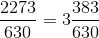### Example Question #2 : Decimals With Fractions

Simplify: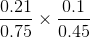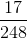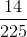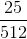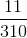Explanation:

1. Multiply the two fractions together in decimal form: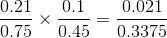2. Multiply the new fraction by 10,000/10,000: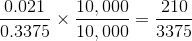3. Simplify this fraction using the greatest common factor:

In this case the GCF is 15: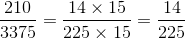### Example Question #3 : Decimals With Fractions

What is the fraction value of 0.833?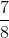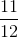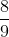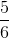Explanation: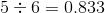### Example Question #4 : Decimals With Fractions

A '95 Chevy Silverado acheives maximum fuel efficiency on a level highway and ideal road conditions at 25 mpg. Driving in the city, the fuel efficiency decreases to about 15 mpg. What is the percent fuel efficiency the truck has in city driving conditions?

75%

60%

80%

40%

50%

60%

Explanation:

Finding the % fuel efficiency for city driving starts with setting up a fraction with the maximum niles per gallon as your denominator and your actual mpg as your numerator: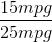This fraction can be simplified into a decimal: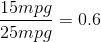Which, when multiplied by 100% will give you your % fuel efficiency:

0.6 x 100% = 60%

### Example Question #5 : Decimals With Fractions

Wind energy is big business in countries like the Netherlands. If a wind turbine is designed to spin at a maximum rate of 250 rotations per minute (RPM) but due to large trees and other obstructions, the mill only spins at 185 RPM, what is the percent efficiency?

82%

67%

74%

78%

92%

74%

Explanation: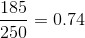0.74 x 100% = 74% Efficiency

### Example Question #6 : Decimals With Fractions

What is the fraction value of the decimal 0.11?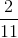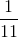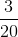Explanation:

If we were to divide 1 by the decimal value we get: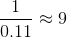From this we can determine that 1 is our numerator and 9 is our denominator, giving us:### Example Question #7 : Decimals With Fractions

There are 200 parking spaces at the local shopping mall and a decimal value of 0.85 (1.0 being 100% of spaces) of the spaces are occupied by parked cars. What is the simplified fraction value of the proportion of spaces that area still available?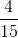Explanation:

Since 0.85 (85%) of the spaces are occupied, we can conclude that 0.15 (15%) are vacant. We can calculate the number of available spaces by multipling the decimal by the total number of spaces: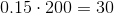To get a fraction value, we put the number of vacant spaces as the numerator and the total number of spaces as the denominator and begin to simplify: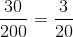### Example Question #8 : Decimals With Fractions

What is the decimal value for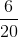?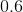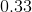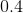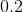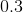Explanation: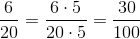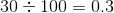### Example Question #9 : Decimals With Fractions

There is a high proportion of people who work in investment banking who are also involved with fraud. Industry specialists place the figure at a decimal value of 0.05. If a firm hires 200 stock brokers, how many of them are likely involved with fraudulent schemes?Explanation:

The decimal value 0.05 can be put into fraction form as such: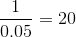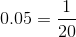Now that we know the fraction proportion of fraudulent investors, we can determine how many of the 200 employed may be guilty of fraud: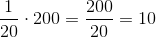### Example Question #10 : Decimals With Fractions

What is the decimal value of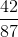?